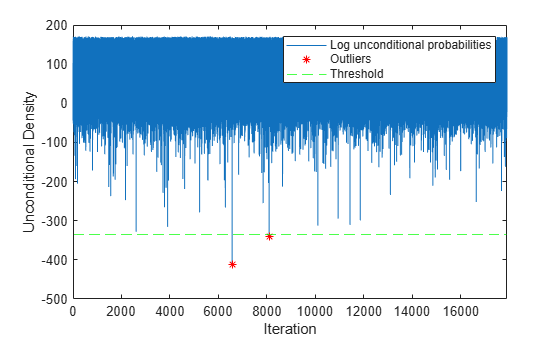# logp

Log unconditional probability density of naive Bayes classification model for incremental learning

Since R2021a

## Syntax

``lp = logp(Mdl,X)``

## Description

example

````lp = logp(Mdl,X)` returns the log unconditional probability densities `lp` of the observations in the predictor data `X` using the naive Bayes classification model for incremental learning `Mdl`. You can use `lp` to identify outliers in the training data.```

## Examples

collapse all

Train a naive Bayes classification model by using `fitcnb`, convert it to an incremental learner, and then use the incremental model to detect outliers in streaming data.

Load the human activity data set. Randomly shuffle the data.

```load humanactivity rng(1); % For reproducibility n = numel(actid); idx = randsample(n,n); X = feat(idx,:); Y = actid(idx);```

For details on the data set, enter `Description` at the command line.

Train Naive Bayes Classification Model

Fit a naive Bayes classification model to a random sample of about 25% of the data.

```idxtt = randsample([true false false false],n,true); TTMdl = fitcnb(X(idxtt,:),Y(idxtt))```
```TTMdl = ClassificationNaiveBayes ResponseName: 'Y' CategoricalPredictors: [] ClassNames: [1 2 3 4 5] ScoreTransform: 'none' NumObservations: 6167 DistributionNames: {1x60 cell} DistributionParameters: {5x60 cell} Properties, Methods ```

`TTMdl` is a `ClassificationNaiveBayes` model object representing a traditionally trained model.

Convert Trained Model

Convert the traditionally trained model to a naive Bayes classification model for incremental learning.

`IncrementalMdl = incrementalLearner(TTMdl)`
```IncrementalMdl = incrementalClassificationNaiveBayes IsWarm: 1 Metrics: [1x2 table] ClassNames: [1 2 3 4 5] ScoreTransform: 'none' DistributionNames: {1x60 cell} DistributionParameters: {5x60 cell} Properties, Methods ```

`IncrementalMdl` is an `incrementalClassificationNaiveBayes` object. `IncrementalMdl` represents a naive Bayes classification model for incremental learning; the parameter values are the same as the parameters in `TTMdl`.

Detect Outliers

Determine an unconditional density threshold for outliers by using the traditionally trained model and training data. Outliers are observations in the streaming data that yield densities lower than the threshold.

```ttlp = logp(TTMdl,X(idxtt,:)); [~,lower] = isoutlier(ttlp)```
```lower = -336.0424 ```

Detect these outliers in the rest of the data. Simulate a data stream by processing 1 observation at a time. At each iteration, call `logp` to compute the log unconditional probability density of the observation and store each value.

```% Preallocation idxil = ~idxtt; nil = sum(idxil); numObsPerChunk = 1; nchunk = floor(nil/numObsPerChunk); lp = zeros(nchunk,1); iso = false(nchunk,1); Xil = X(idxil,:); Yil = Y(idxil); % Incremental processing for j = 1:nchunk ibegin = min(nil,numObsPerChunk*(j-1) + 1); iend = min(nil,numObsPerChunk*j); idx = ibegin:iend; lp(j) = logp(IncrementalMdl,Xil(idx,:)); iso(j) = lp(j) < lower; end```

Plot the log unconditional probability densities of the streaming data. Identify the outliers.

```figure; h1 = plot(lp); hold on x = 1:nchunk; h2 = plot(x(iso),lp(iso),'r*'); h3 = yline(lower,'g--'); xlim([0 nchunk]); ylabel('Unconditional Density') xlabel('Iteration') legend([h1 h2 h3],["Log unconditional probabilities" "Outliers" "Threshold"]) hold off```## Input Arguments

collapse all

Naive Bayes classification model for incremental learning, specified as an `incrementalClassificationNaiveBayes` model object. You can create `Mdl` directly or by converting a supported, traditionally trained machine learning model using the `incrementalLearner` function. For more details, see the corresponding reference page.

You must configure `Mdl` to compute the log conditional probability densities on a batch of observations.

• If `Mdl` is a converted, traditionally trained model, you can compute the log conditional probabilities without any modifications.

• Otherwise, `Mdl.DistributionParameters` must be a cell matrix with `Mdl.NumPredictors` > 0 columns and at least one row, where each row corresponds to each class name in `Mdl.ClassNames`.

Batch of predictor data with which to compute the log conditional probability densities, specified as an n-by-`Mdl.NumPredictors` floating-point matrix.

For each `j` = 1 through n, if `X(j,:)` contains at least one `NaN`, `lp(j)` is `NaN`.

Data Types: `single` | `double`

## Output Arguments

collapse all

Log unconditional probability densities, returned as an n-by-1 floating-point vector. `lp(j)` is the log unconditional probability density of the predictors evaluated at `X(j,:)`.

Data Types: `single` | `double`

collapse all

### Unconditional Probability Density

The unconditional probability density of the predictors is the density's distribution marginalized over the classes.

In other words, the unconditional probability density is

`$P\left({X}_{1},..,{X}_{P}\right)=\sum _{k=1}^{K}P\left({X}_{1},..,{X}_{P},Y=k\right)=\sum _{k=1}^{K}P\left({X}_{1},..,{X}_{P}|y=k\right)\pi \left(Y=k\right),$`

where π(Y = k) is the class prior probability. The conditional distribution of the data given the class (P(X1,..,XP|y = k)) and the class prior probability distributions are training options (that is, you specify them when training the classifier).

### Prior Probability

The prior probability of a class is the assumed relative frequency with which observations from that class occur in a population.

## Version History

Introduced in R2021a